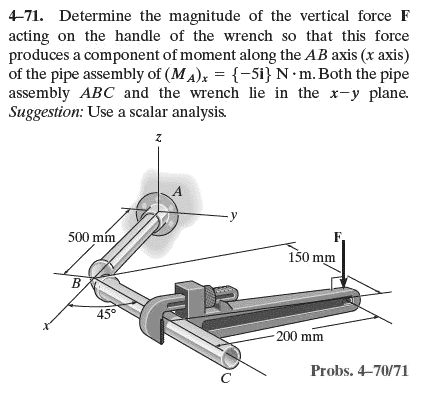# Statics - Moment using scalar analysis

## Homework Statement## Homework Equations

I think that I need to find the moment of the force F about the line BC first.

Then, using that moment, find the "projection" of $M_{BC}$ onto the X axis to find the answer. $M_{BC}\sin \left(45^{o}\right)$

However, I am not getting anything near the solution value.

## The Attempt at a Solution

My only attempt at solving this that came close to correct was to use the distance from point B to F. That was obviously wrong (but yielded 20 lb - which was extremely close to the 20.2 given by the author.)

(I know that is wrong...) As soon as I sat the problem down to go to the next one, I immediately realized my method was incorrect, but I have tried and tried to get a correct answer and nothing has yielded in 1.5 hrs, so I digress.

I have a test this afternoon, so I cannot waste a lot of time on one that is holding me up. Two more chapters to review...

Any hints will be great!

TIA

The moment of a force about the x axis is $$F_zy + F_yz$$. Since only F_z is acting, there is no F_y term, so you just have to compute the y distance (the perpendicular distance from the x axis to the line of action of the force). You need to watch your geometry and trig to get the corect y value which yields the correct answer of F = 20.2 N.m.